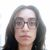# New to Qlik Sense

If you’re new to Qlik Sense, start with this Discussion Board and get up-to-speed quickly.

Announcements
QlikWorld 2023, a live, in-person thrill ride. Save \$300 before February 6: REGISTER NOW!
cancel
Showing results for
Did you mean:Partner - Contributor III

## Set analysis sum until last month

Hi,

I have a problem with set analysis. I need to calculate the balances for the different months:

January                                                                              February                                                                              March

Initial balance=10  (of the previous year)            Initial balance=12 (10+2)                                     Initial balance=15 (10+2+3)

Payments/charges=+2                                           Payments/charges=+3                                                    Payments/charges=+1

Final balance=12                                                      Final balance=15 (10+2+3)                                       Final balance=16 (10+2+3+1)

So, I am trying to use this expression for the initial balance:

if(Month='ene.'
,sum(\${<Year={'\$(v_MaxAnio)'}>}Saldo_ej_anterior), sum({<MonthNum={">=1<\$(v_MaxMes)"},Year={'\$(v_MaxAnio)'}>}Saldo) +sum({\$<Year={'\$(v_MaxAnio)'}>}Saldo_ej_anterior))

Where MaxMes=     =Num(month(Max(Date)))

MaxAnio=   max([Year Month Text])

saldo ej anterior=balance of the previous year

Saldo=balance

When a month is not selected this expresssion: sum({<MonthNum={">=1<\$(v_MaxMes)"},Year={'\$(v_MaxAnio)'}>}Saldo) works fine ( it gives me +5(2+3)) but when I select a month, for example, february (i need to use a pivotant table) it gives me only +3 and I need the sum of the previous months (+2, for the initial balance) and the sum of all the months (+5, for the final balance).

I could really use your help because I am desperate with this.

0 RepliesTags
Community Browser